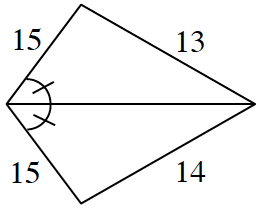### Home > INT1 > Chapter 8 > Lesson 8.2.1 > Problem8-102

8-102.

Carefully examine each diagram below and explain why the geometric figure cannot exist. Support your statements with reasons.1.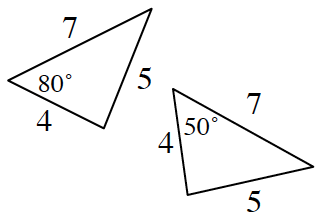Read the Math Notes box from Lesson 7.1.6 to review the triangle congruence conjectures.

The triangles should be congruent by $\text{SSS} ≅$, but $80º ≠ 50º$ and in congruent triangles all angles are equal as well.

1.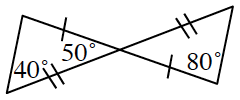The triangles should be congruent by $\text{SAS} ≅$, but $80º ≠ 90º$ and $40º ≠ 50º$ and in congruent triangles all angles are equal as well.

1.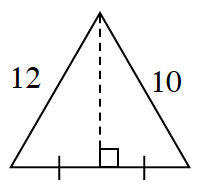The triangles should be congruent by $\text{SAS} ≅$, but $10 ≠ 12$ and in congruent triangles all sides are congruent as well.

1.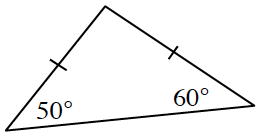What do you know about isosceles triangles?

1.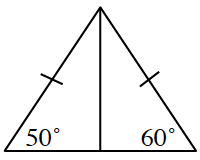1.